Want to share your content on R-bloggers? click here if you have a blog, or here if you don't.

## Background

I was honoured by a site visit by Drs. Yeo-Min Yun and Junghan Song of the Korean Society for Clinical Chemistry a few weeks ago. As both professors are on the organizing committee of the Cherry Blossom Symposium for Lab Automation in Seoul in Spring 2016, their primary motivation for visiting was to discuss mass spectrometry sample prep automation but later we got on to the topic of automated reporting for quality assurance schemes.

Naturally, I was promoting R, R-Markdown and knitr as a good pipeline for automated Quality Assurance (QA) report.

This brought to mind Youden Plots which are used by DGKL in their reports. I like DGKL reports for three reasons:

1. They are accuracy based against GC-MS when it comes to steroids.

2. I can see all the other LC-MS/MS methods immediately.

3. Youden plots look like a target and can be assessed rapidly.

The data for a Youden plot is generated by providing a number of laboratories aliquots from two separate unknown samples, which we will call A and B. Every lab analyzes both samples and a scatter plot of the A and B results are generated–the A results on the (x)–axis and the B results on the (y)–axis. Once this is completed, limits of acceptability are plotted and outliers can be identified.

In Youden's original formulation of the plot (see page 133-1 this online document) he required that the concentrations of the A and B samples be close to one another. As you might guess, in clinical medicine, this is not all that useful because we often want to test more than one part of the analytical range in an external quality assurance (EQA) scheme. One workaround for this is the make a Youden plot of the standard normal variates for the A and B samples, that is to plot (z_b = frac{b_i-bar{b}} {sigma_b}) vs (z_a = frac{a_i-bar{a}} {sigma_a}), where (a_i) and (b_i) are the individual values of the A and B samples from the (i) labs. This has the disadvantage of representing the results in a manner that is not easily assessed from a clinical perspective.

While there are published approaches to coping with this problem, these are out of scope here but I will show you a couple of other ways I have seen Youden plots represented. If you want to see R code to generate the classic Youden Plot, it can be found in this this stackoverflow post and below.

## Random Data

Let's start by generating some data. For the sake of argument, let's say we are looking at testosterone results in males and measured in nmol/L. Suppose that the A sample has a true concentration of 5.3 nmol/L and the B sample has a true concentration of 16.2 nmol/L. Let's also assume that they are all performed by the same analytical method. If you have looked at EQA reports, you will know that a scatter plot of results for the A and B samples does not typically look like this.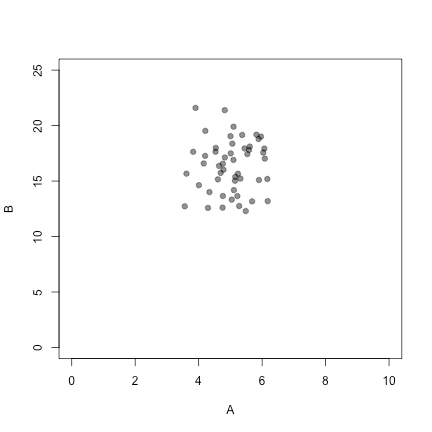The (mock) data abaove are bivariate Gaussian and uncorrelated. In reality we often see something that looks a little more like this: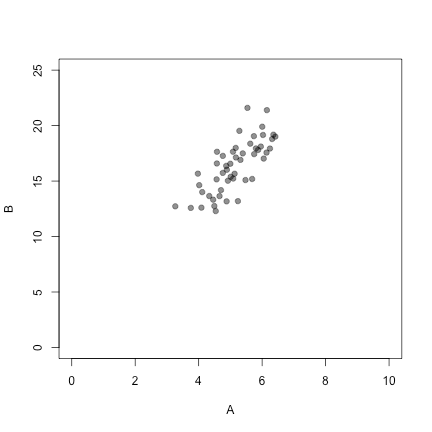That is, the A and B values are usually correlated.

## Rectangular Youden Plots

The most common manner in which you will see a Youden plot prepared is just a box with mean (pm) 2SD and (pm) 3SD limits.

```plot(A,B, xlim = c(0,10), ylim = c(0,30), pch=19, col="#00000080")
grid()

A.mu <- mean(A)
A.sd <- sd(A)
B.mu <- mean(B)
B.sd <- sd(B)

#draw a box around the 2SD limit
rect(xleft = A.mu - 2*A.sd, ybottom = B.mu - 2*B.sd, xright = A.mu + 2*A.sd, ytop = B.mu + 2*B.sd, lwd = 2, border = "orange")
#draw a box around the 3SD limit
rect(xleft = A.mu - 3*A.sd, ybottom = B.mu - 3*B.sd, xright = A.mu + 3*A.sd, ytop = B.mu + 3*B.sd, lwd = 2, border = "red")

#draw a diagonal line - which is unnecessary but you will see people do it
lines(c(A.mu - 3*A.sd, A.mu + 3*A.sd),c(B.mu - 3*B.sd, B.mu + 3*B.sd))
#draw horizontal line
lines(c(A.mu - 3*A.sd, A.mu + 3*A.sd), c(B.mu, B.mu))
#draw vertical line
lines(c(A.mu, A.mu), c(B.mu - 3*B.sd, B.mu + 3*B.sd))

legend("topleft", c("2SD limit","3SD limit"), col=c("orange","red"), lty=c(1,1))```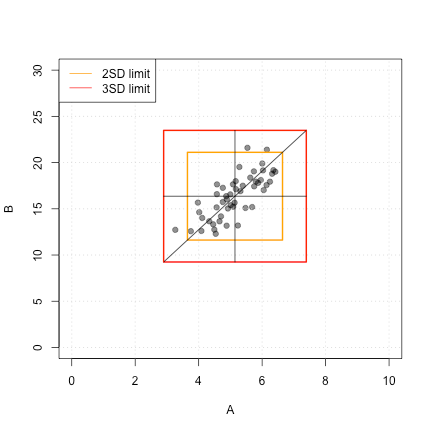## Non Parametric

Obviously, if you prefer, you could prepare this Youden plot in a non-parametric fashion by plotting the medians and the non-parametrically calculated 1st, 2.5th, 97.5th, and 99th percentiles. In this case, the code would be:

```plot(A,B, xlim = c(0,10), ylim = c(0,30), pch=19, col="#00000080")
grid()

A.med <- median(A)
A.quants <- quantile(A, probs=c(0.01,0.025,0.975,0.99))
B.med <- median(B)
B.quants <- quantile(B, probs=c(0.01,0.025,0.975,0.99))

#draw a box around the central 95% limit
rect(xleft = A.quants, ybottom = B.quants, xright = A.quants, ytop = B.quants, lwd = 2, border = "orange")
#draw a box around the central 99% limit
rect(xleft = A.quants, ybottom = B.quants, xright = A.quants, ytop = B.quants, lwd = 2, border = "red")

#draw a vertical line
lines(c(A.quants,A.quants),c(B.med,B.med))
#draw vertical line
lines(c(A.med,A.med),c(B.quants,B.quants))

legend("topleft", c("Central 95%","Central 99%"), col=c("orange","red"), lty=c(1,1))```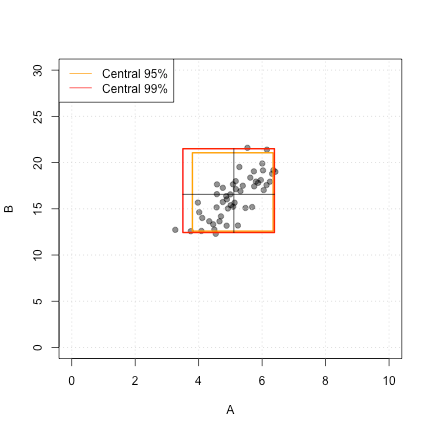Note that if you increase the number of points, this non-parametric plot will not quite converge to look like the parametric one shown above because (mu pm 2sigma) actually encompases 95.45% of the data in a univariate normal distribution, and (mu pm 3sigma) actually encompases 99.73% of the data.

## FYI

Be ye not deceived. Even with the non-parametric approach or a parametric approach with the correct (z)-scores, the orange (“Central 95%” or “2SD”) boxes shown above do not house 95% of the data and the red (“Central 99%” or “3SD”) boxes do not house 99% of the data. You can see this pretty easily if you consider the case of uncorrelated data. Let's take 100000 random pairs of uncorrelated Gaussian data with (mu = 10) and (sigma = 1).

Five percent of data points are excluded by the vertical orange lines shown below at the (mu_A pm 1.96 sigma_A) and 5% of data are excluded by the horizontal orange lines positioned at (mu_B pm 1.96 sigma_B).

Points will fall into the one of the 4 areas shaded yellow 0.95 x 0.025 or 2.375 % of the time and points will fall into one of the 4 areas shaded purple 0.025 x 0.025 or 0.0625 % of the time. This means that the “2SD” box actually encloses 100 – 4×2.375 – 4×0.0625 % = 90.25 % of the data.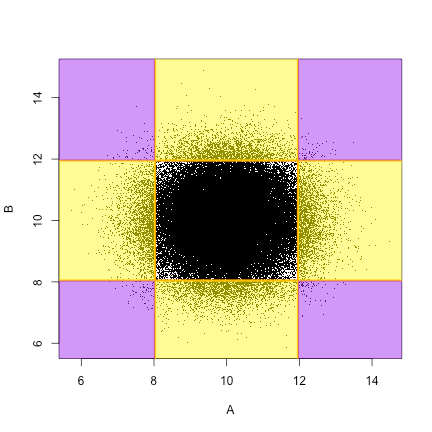Much more directly, the probability of both A and B falling inside the center square is 0.95×0.95 = 0.9025 = 90.25%.

You can do a random simulation to prove this to yourself:

```#generate the data, n=10000
A <- rnorm(10^5,10,1)
B <- rnorm(10^5,10,1)
# convert results to z-scores
scale.df <- data.frame(scA = scale(A), scB = scale(B))
#calculate how many samples are inside the centre box
nrow(subset(scale.df, abs(scA)<1.96 & abs(scB)<1.96))```

`##  90245`

This is pretty darn close to the 90.25% we were expecting.

## Elliptical Youden Plots

The rectangular plot shown works (with caveats described) but there is something slightly undesirable about it because a point could be off in the corner, far away from the other data, but still inside the 3SD box. It seems much preferable to encircle the data with an ellipse. Fortunately, there is a built in function to achieve this in the `car` package which makes the code is very simple. The other nice thing is that the ellipses are actually calculated to house 95% and 99% of the data respectively.

```library(car)
dataEllipse(A, B, levels=c(0.95, 0.99), fill = FALSE, xlim = c(0,10), ylim = c(0,30), pch = 19, col=c("#00000080", "red"), center.pch = FALSE)```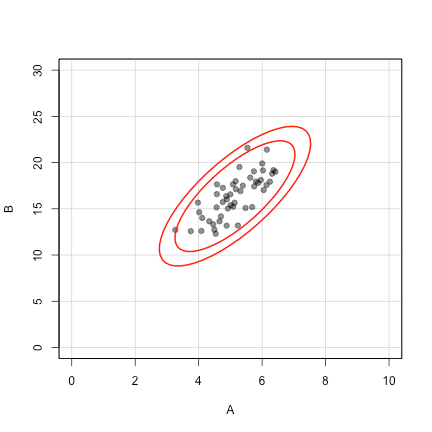To generate a two–colour equivalent to what we have above we draw the Youden plot in two stages.

```dataEllipse(A, B, levels = 0.95, fill = FALSE, xlim = c(0,10), ylim = c(0,30), pch = 19, col = c("#00000080", "orange"), center.pch = FALSE)

dataEllipse(A, B, levels = 0.99, fill = FALSE, col = c(NA, "red"), center.pch = FALSE, plot.points = FALSE)

legend("topleft", c("Central 95%","Central 99%"), col = c("orange","red"), lty=c(1,1))```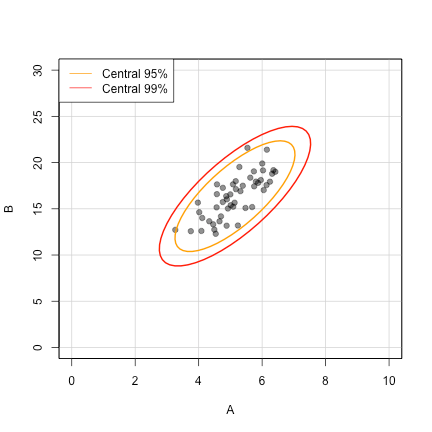Now we might want to add the horizontal, vertical lines.

```#store the points of the outer ellipse in a matrix
outer.ellipse <- dataEllipse(A, B, levels = 0.99, xlim = c(0,10), ylim = c(0,30), pch = 19, col = c("#00000080", "red"), draw = TRUE, center.pch = FALSE)
#plot the innter ellipse
dataEllipse(A, B, levels = 0.95, fill = FALSE, xlim = c(0,10), ylim = c(0,30), pch = 19, col = c("#00000080", "orange"), center.pch = FALSE, plot.points = FALSE, add = TRUE)

#find the value of x that is closest to the mean value of A
vert.index <- which.min(abs(A.mu-outer.ellipse[,1]))
#calculate the vertical distance to centre
vert.dist <- outer.ellipse[,2][vert.index] - B.mu
#draw the line one end of ellipse to the other
lines(c(A.mu, A.mu), c(outer.ellipse[,2][vert.index], outer.ellipse[,2][vert.index] - 2*vert.dist))

#find the value of y that is closest to the mean valye of B
horiz.index <- which.min(abs(B.mu-outer.ellipse[,2]))
#calculate the horizonal distance to centre
horiz.dist <- outer.ellipse[,1][horiz.index] - A.mu
#draw the line one end of ellipse to the other
lines(c(outer.ellipse[,1][horiz.index], outer.ellipse[,1][horiz.index] - 2*horiz.dist), c(B.mu,B.mu))
legend("topleft", c("Central 95%","Central 99%"), col=c("orange","red"), lty=c(1,1))```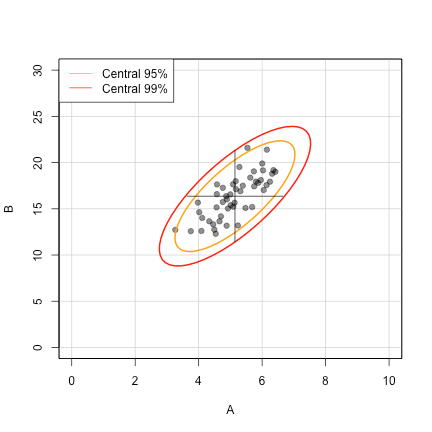And if you wanted a little more groove you can add shading.

```dataEllipse(A, B, levels = 0.99, xlim = c(0,10), ylim = c(0,30), pch = 19, col = c("#00000080", "red"), center.pch = FALSE, plot.points = TRUE, fill = TRUE, fill.alpha = 0.1)
dataEllipse(A, B, levels=0.95 ,xlim = c(0,10), ylim = c(0,30), pch = 19, col="orange", center.pch = FALSE, plot.points = FALSE, add = TRUE, fill = TRUE, fill.alpha = 0.3)
lines(c(A.mu, A.mu), c(outer.ellipse[,2][vert.index], outer.ellipse[,2][vert.index] - 2*vert.dist), col = "blue")
lines(c(outer.ellipse[,1][horiz.index], outer.ellipse[,1][horiz.index] - 2*horiz.dist), c(B.mu,B.mu), col = "blue")
legend("topleft", c("Central 95%","Central 99%"), col=c("orange","red"), lty=c(1,1))```## Build a Function

We can also fold all of this into a function:

```youden <- function(A,B,shape){

A.mu <- mean(A)
A.sd <- sd(A)
B.mu <- mean(B)
B.sd <- sd(B)

plot(A,B, pch=19, col = "#00000080", xlim = c(A.mu - 5*A.sd,A.mu + 5*A.sd), ylim = c(B.mu - 5*B.sd,B.mu + 5*B.sd))
grid()

if (missing(shape)){
shape <- "ellipse"
}

if (shape == "rectangle"){
rect(xleft = A.mu - 2*A.sd, ybottom = B.mu - 2*B.sd, xright = A.mu + 2*A.sd, ytop = B.mu + 2*B.sd, lwd = 2, border = "orange")
rect(xleft = A.mu - 3*A.sd, ybottom = B.mu - 3*B.sd, xright = A.mu + 3*A.sd, ytop = B.mu + 3*B.sd, lwd = 2, border = "red")
lines(c(A.mu - 3*A.sd, A.mu + 3*A.sd),c(B.mu - 3*B.sd, B.mu + 3*B.sd))
lines(c(A.mu - 3*A.sd, A.mu + 3*A.sd), c(B.mu, B.mu))
lines(c(A.mu, A.mu), c(B.mu - 3*B.sd, B.mu + 3*B.sd))
legend("topleft", c("2SD limit","3SD limit"), col=c("orange","red"), lty=c(1,1))

} else if (shape=="ellipse") {
outer.ellipse <- dataEllipse(A, B, levels = 0.99, pch = 19, col=c("#00000080", "red"), draw = TRUE, center.pch = FALSE, plot.points = FALSE)
dataEllipse(A, B, levels = 0.95, fill = FALSE, pch = 19, col=c("#00000080", "orange"), center.pch = FALSE, plot.points = FALSE, add = TRUE)
vert.index <- which.min(abs(A.mu-outer.ellipse[,1]))
vert.dist <- outer.ellipse[,2][vert.index] - B.mu
lines(c(A.mu, A.mu), c(outer.ellipse[,2][vert.index], outer.ellipse[,2][vert.index] - 2*vert.dist))
horiz.index <- which.min(abs(B.mu-outer.ellipse[,2]))
horiz.dist <- outer.ellipse[,1][horiz.index] - A.mu
lines(c(outer.ellipse[,1][horiz.index], outer.ellipse[,1][horiz.index] - 2*horiz.dist), c(B.mu,B.mu))
legend("topleft", c("Central 95%","Central 99%"), col=c("orange","red"), lty=c(1,1))
}
}

#use the function
par(mfrow=c(1,2))
youden(A,B, shape = "rectangle")
youden(A,B, shape = "ellipse")```## Comparison with the Classic Youden Plot

If the data happens to be uncorrelated, and has identical average A and B values, the elliptical approach will generate a (nearly) circular Youden plot according to the original description. The `youden.classic()` function code shown below is borrowed from the stackoverflow post mentioned above.

```youden.classic <- function(A, B){
plot(A,B,asp = 1, xlab = "A", ylab = "B", pch=".")
mB <- median(B)
mA <- median(A)
abline(h = mB, v = mA)
d <- mean(A-B)
d_prime <- A-B-d
r <- 2.45*mean(abs(d_prime))*sqrt(pi)/2
t <- seq(0,2*pi,by=0.01)
x <- r*cos(t)+mA
y <- r*sin(t)+mB
lines(x,y)
}

#generate 10000 pairs of bivariate data with a mean of 10 for both A and B and 15% CV
A <- rnorm(10000,10,1.5)
B <- rnorm(10000,10,1.5)
youden.classic(A,B)

#overlay ellipse
dataEllipse(A, B, levels = 0.95, fill = TRUE, fill.alpha = 0.3, col="orange", center.pch = FALSE, plot.points = FALSE, add = TRUE)```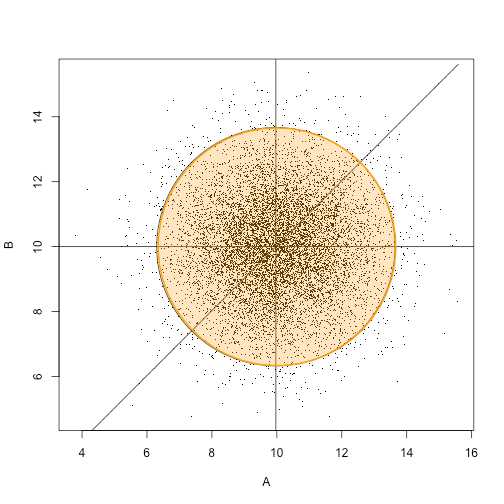## Conclusion

There you have it. With a Youden Plot it is easy to separate the sheep from the goats. There are lots of ways that you can dress up your plot to suit your needs. Of course, this could be embedded into an automated EQA report generated with R, Rmarkdown and knitr.